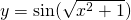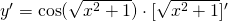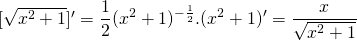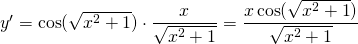# Solution assignment 23 Chain rule

### Assignment 23

Differentiate:### Solution

This function requires a double chain rule as we will see (the 'quote' means: the derivative of):First we calculate the second factor, again using the chain rule:We can substitute this result in the derivative above: